Publicité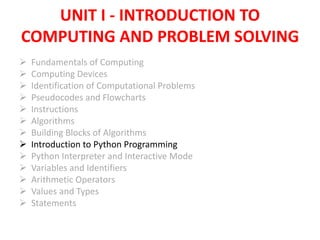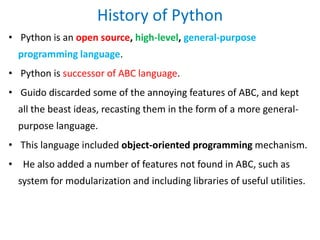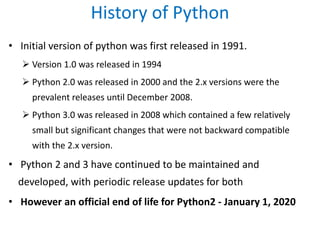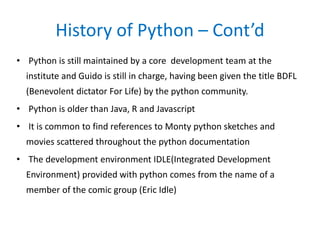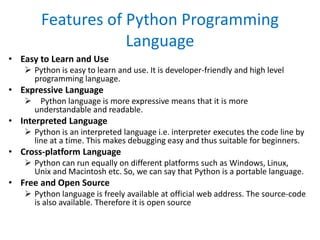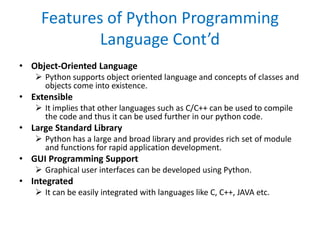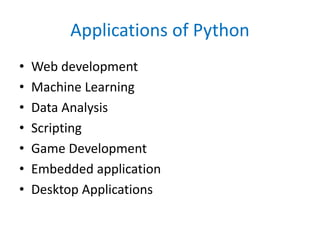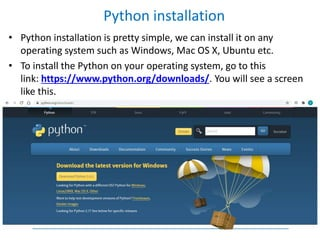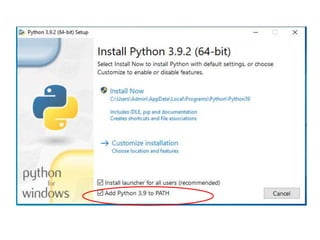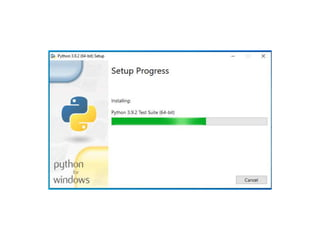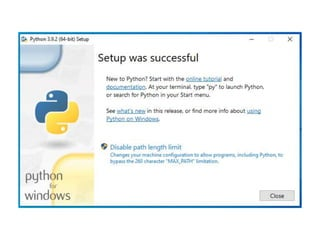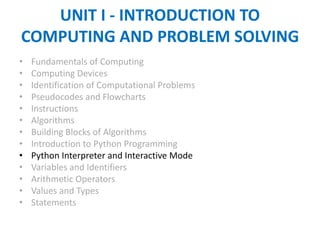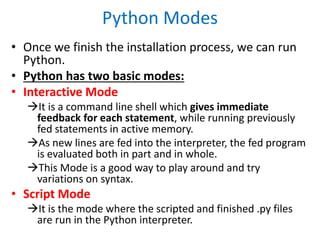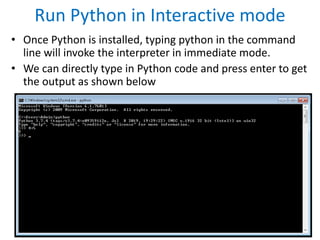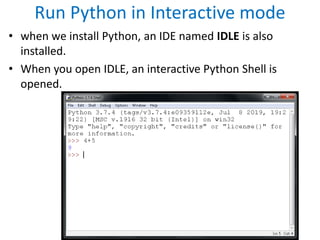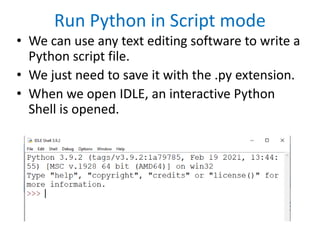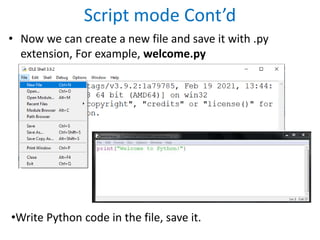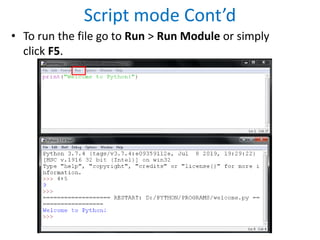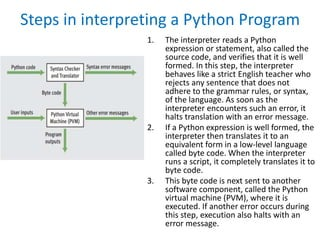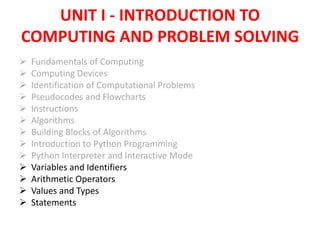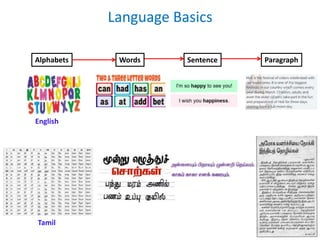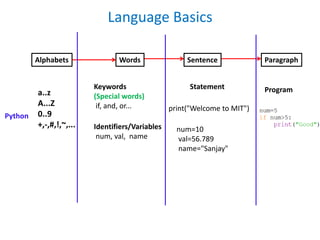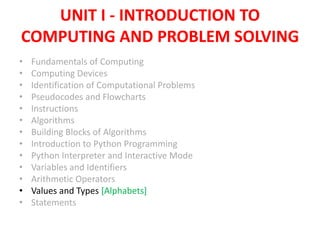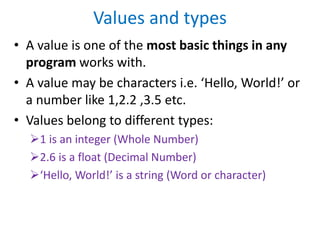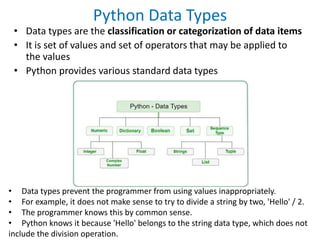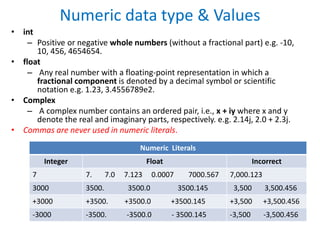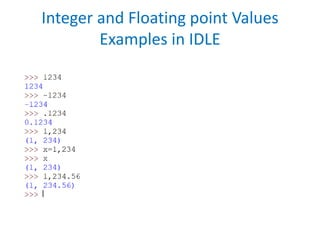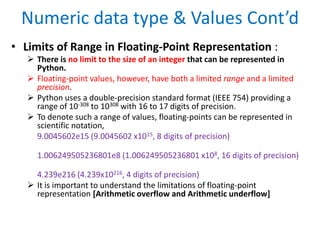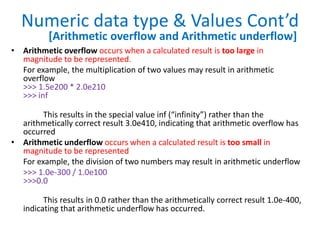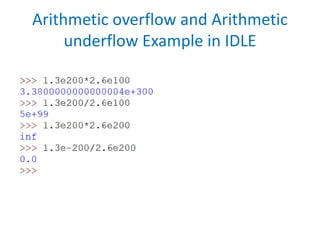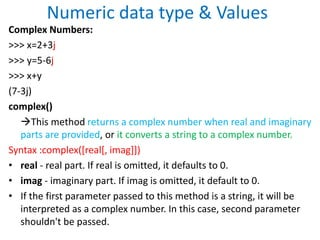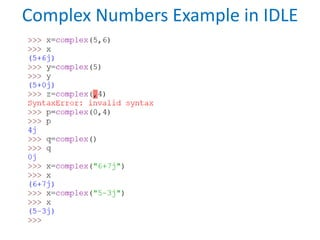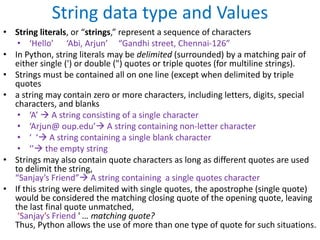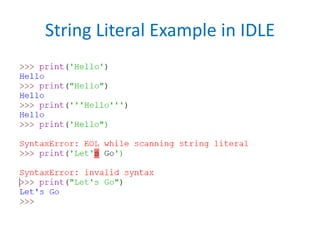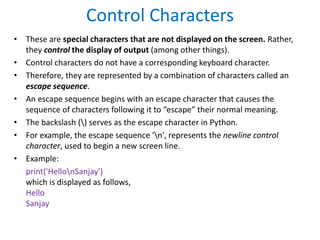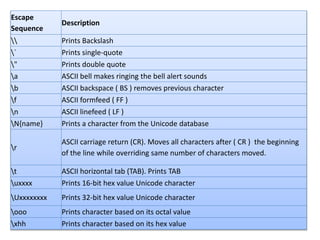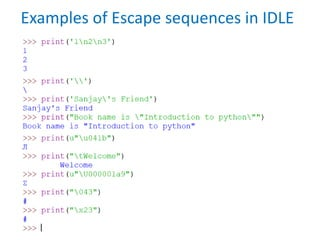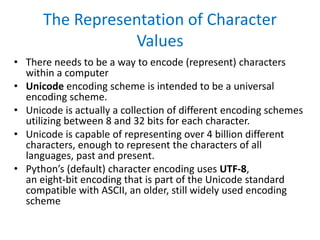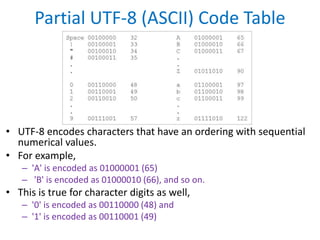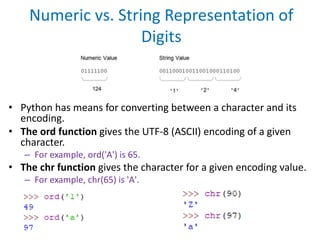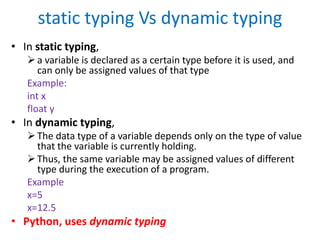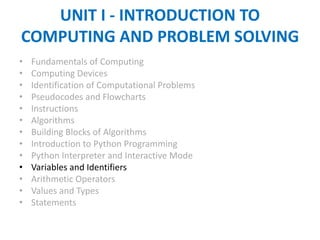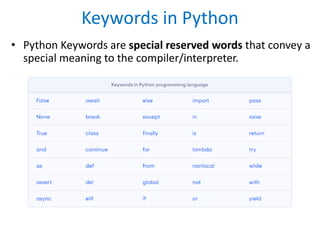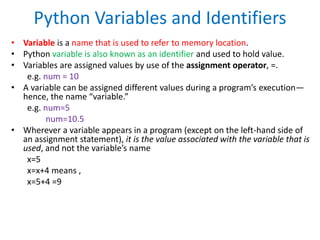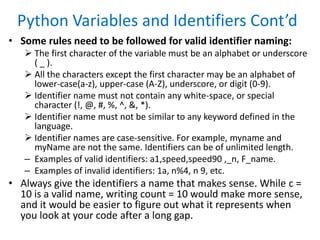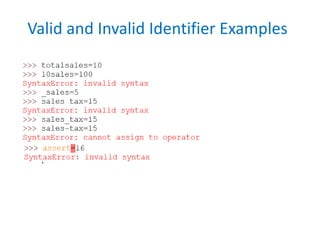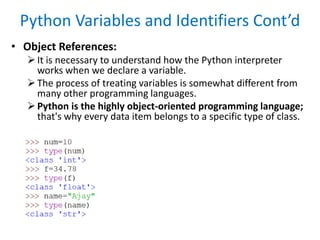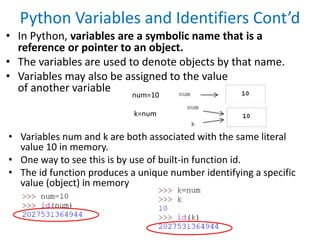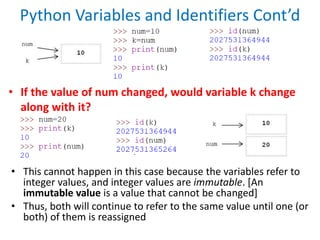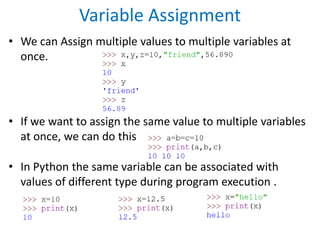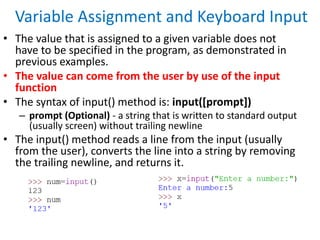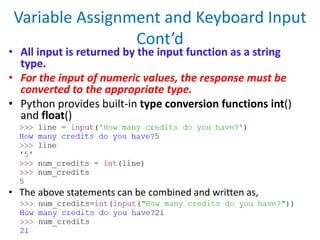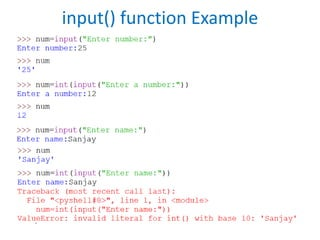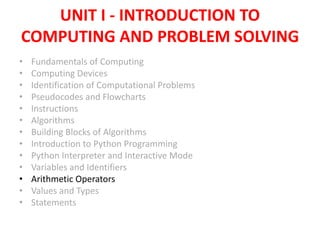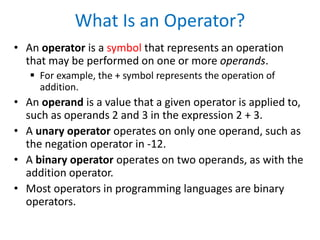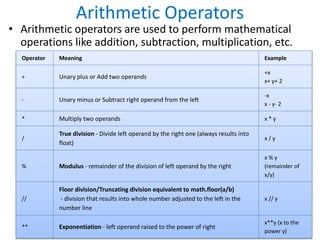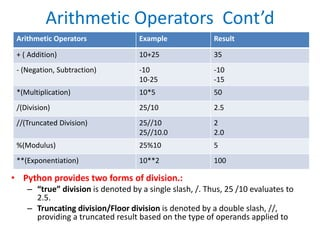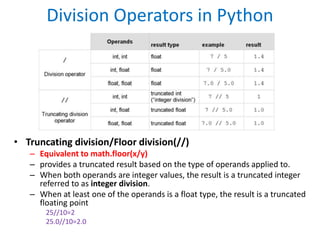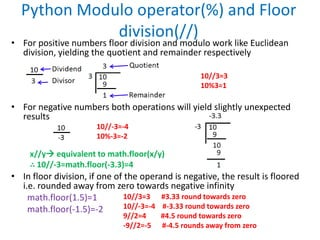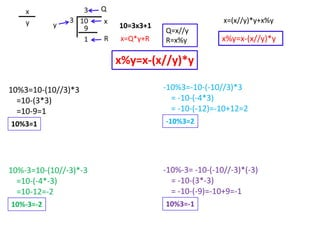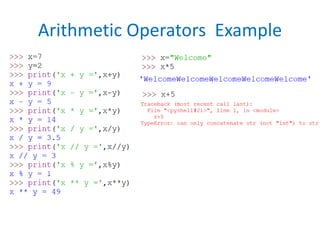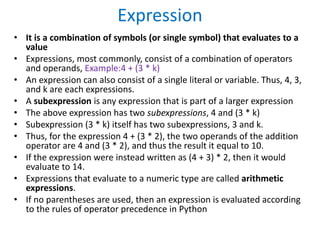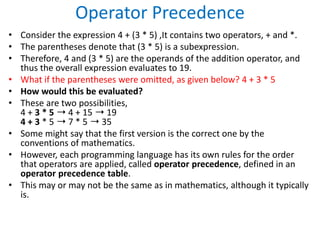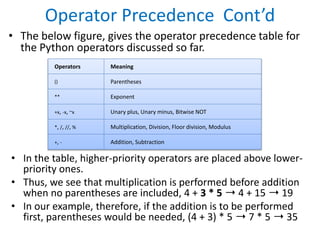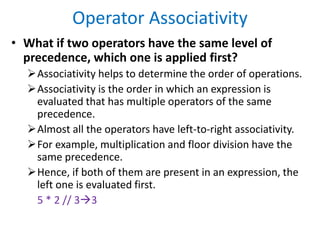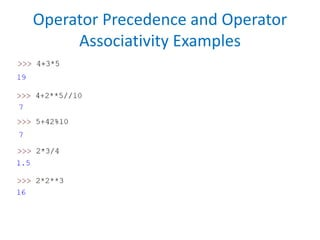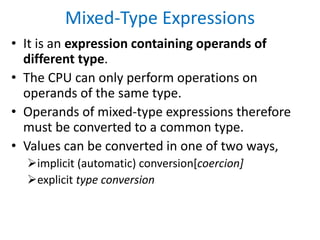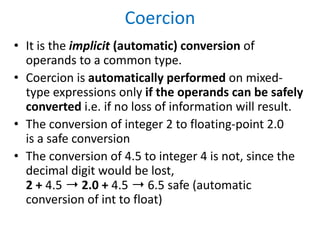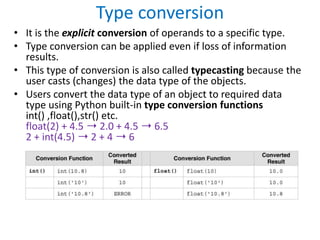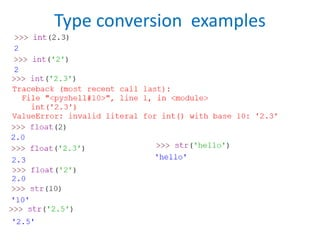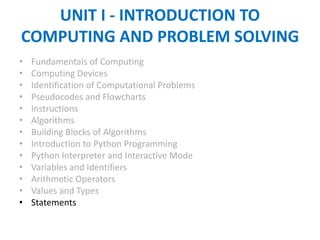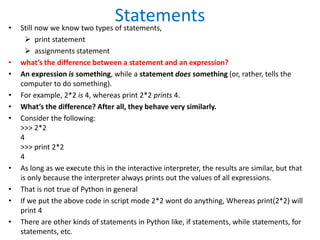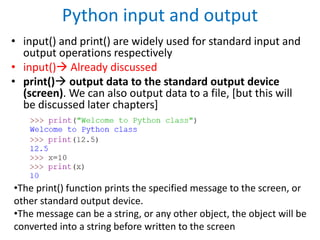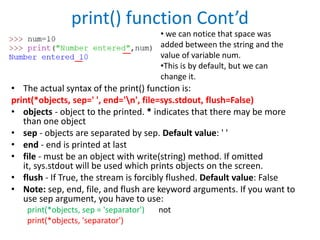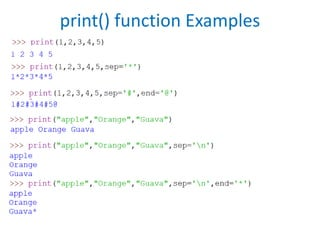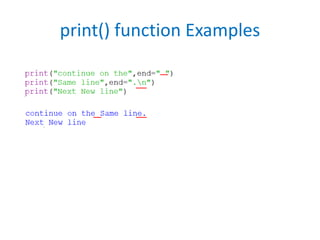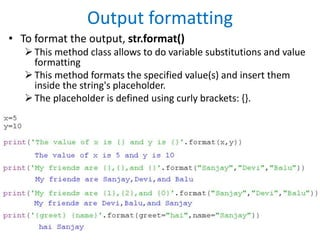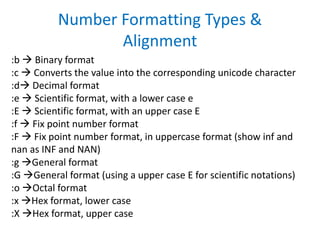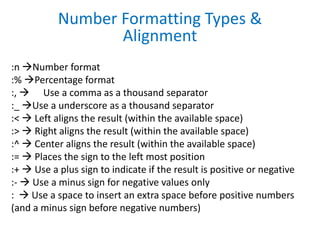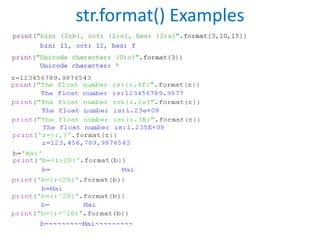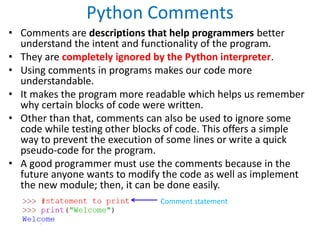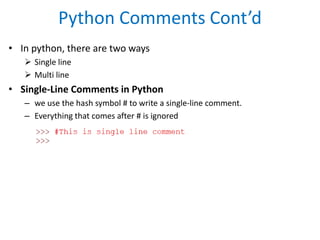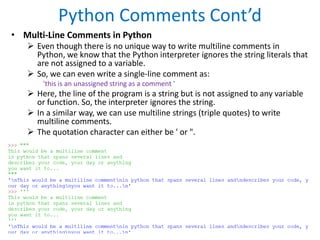1 sur 85
Publicité

### 4_Introduction to Python Programming.pptx

1. UNIT I - INTRODUCTION TO COMPUTING AND PROBLEM SOLVING  Fundamentals of Computing  Computing Devices  Identification of Computational Problems  Pseudocodes and Flowcharts  Instructions  Algorithms  Building Blocks of Algorithms  Introduction to Python Programming  Python Interpreter and Interactive Mode  Variables and Identifiers  Arithmetic Operators  Values and Types  Statements
2. Introduction to Python Programming • Python was developed by Guido van Rossum in late 1980s at the National Research Institute for Mathematics and Computer Science in Netherlands. • Guido named it after the Television show “Monty Python’s flying circus”, of which Guido was, and presumably still is, a fan.
3. History of Python • Python is an open source, high-level, general-purpose programming language. • Python is successor of ABC language. • Guido discarded some of the annoying features of ABC, and kept all the beast ideas, recasting them in the form of a more general- purpose language. • This language included object-oriented programming mechanism. • He also added a number of features not found in ABC, such as system for modularization and including libraries of useful utilities.
4. History of Python • Initial version of python was first released in 1991.  Version 1.0 was released in 1994  Python 2.0 was released in 2000 and the 2.x versions were the prevalent releases until December 2008.  Python 3.0 was released in 2008 which contained a few relatively small but significant changes that were not backward compatible with the 2.x version. • Python 2 and 3 have continued to be maintained and developed, with periodic release updates for both • However an official end of life for Python2 - January 1, 2020
5. History of Python – Cont’d • Python is still maintained by a core development team at the institute and Guido is still in charge, having been given the title BDFL (Benevolent dictator For Life) by the python community. • Python is older than Java, R and Javascript • It is common to find references to Monty python sketches and movies scattered throughout the python documentation • The development environment IDLE(Integrated Development Environment) provided with python comes from the name of a member of the comic group (Eric Idle)
6. Features of Python Programming Language • Easy to Learn and Use  Python is easy to learn and use. It is developer-friendly and high level programming language. • Expressive Language  Python language is more expressive means that it is more understandable and readable. • Interpreted Language  Python is an interpreted language i.e. interpreter executes the code line by line at a time. This makes debugging easy and thus suitable for beginners. • Cross-platform Language  Python can run equally on different platforms such as Windows, Linux, Unix and Macintosh etc. So, we can say that Python is a portable language. • Free and Open Source  Python language is freely available at official web address. The source-code is also available. Therefore it is open source
7. Features of Python Programming Language Cont’d • Object-Oriented Language  Python supports object oriented language and concepts of classes and objects come into existence. • Extensible  It implies that other languages such as C/C++ can be used to compile the code and thus it can be used further in our python code. • Large Standard Library  Python has a large and broad library and provides rich set of module and functions for rapid application development. • GUI Programming Support  Graphical user interfaces can be developed using Python. • Integrated  It can be easily integrated with languages like C, C++, JAVA etc.
8. Applications of Python • Web development • Machine Learning • Data Analysis • Scripting • Game Development • Embedded application • Desktop Applications
9. • Python installation is pretty simple, we can install it on any operating system such as Windows, Mac OS X, Ubuntu etc. • To install the Python on your operating system, go to this link: https://www.python.org/downloads/. You will see a screen like this. Python installation
10. UNIT I - INTRODUCTION TO COMPUTING AND PROBLEM SOLVING • Fundamentals of Computing • Computing Devices • Identification of Computational Problems • Pseudocodes and Flowcharts • Instructions • Algorithms • Building Blocks of Algorithms • Introduction to Python Programming • Python Interpreter and Interactive Mode • Variables and Identifiers • Arithmetic Operators • Values and Types • Statements
11. Python Modes • Once we finish the installation process, we can run Python. • Python has two basic modes: • Interactive Mode It is a command line shell which gives immediate feedback for each statement, while running previously fed statements in active memory. As new lines are fed into the interpreter, the fed program is evaluated both in part and in whole. This Mode is a good way to play around and try variations on syntax. • Script Mode It is the mode where the scripted and finished .py files are run in the Python interpreter.
12. Run Python in Interactive mode • Once Python is installed, typing python in the command line will invoke the interpreter in immediate mode. • We can directly type in Python code and press enter to get the output as shown below
13. Run Python in Interactive mode • when we install Python, an IDE named IDLE is also installed. • When you open IDLE, an interactive Python Shell is opened.
14. Run Python in Script mode • We can use any text editing software to write a Python script file. • We just need to save it with the .py extension. • When we open IDLE, an interactive Python Shell is opened.
15. Script mode Cont’d • Now we can create a new file and save it with .py extension, For example, welcome.py •Write Python code in the file, save it.
16. Script mode Cont’d • To run the file go to Run > Run Module or simply click F5.
17. Steps in interpreting a Python Program 1. The interpreter reads a Python expression or statement, also called the source code, and verifies that it is well formed. In this step, the interpreter behaves like a strict English teacher who rejects any sentence that does not adhere to the grammar rules, or syntax, of the language. As soon as the interpreter encounters such an error, it halts translation with an error message. 2. If a Python expression is well formed, the interpreter then translates it to an equivalent form in a low-level language called byte code. When the interpreter runs a script, it completely translates it to byte code. 3. This byte code is next sent to another software component, called the Python virtual machine (PVM), where it is executed. If another error occurs during this step, execution also halts with an error message.
18. UNIT I - INTRODUCTION TO COMPUTING AND PROBLEM SOLVING  Fundamentals of Computing  Computing Devices  Identification of Computational Problems  Pseudocodes and Flowcharts  Instructions  Algorithms  Building Blocks of Algorithms  Introduction to Python Programming  Python Interpreter and Interactive Mode  Variables and Identifiers  Arithmetic Operators  Values and Types  Statements
19. Alphabets Language Basics Words Sentence Paragraph English Tamil
20. Language Basics Alphabets Words Sentence Paragraph a..z A...Z 0..9 +,-,#,!,~,... Keywords (Special words) if, and, or... Identifiers/Variables num, val, name Python print("Welcome to MIT") num=10 val=56.789 name="Sanjay" Statement Program
21. UNIT I - INTRODUCTION TO COMPUTING AND PROBLEM SOLVING • Fundamentals of Computing • Computing Devices • Identification of Computational Problems • Pseudocodes and Flowcharts • Instructions • Algorithms • Building Blocks of Algorithms • Introduction to Python Programming • Python Interpreter and Interactive Mode • Variables and Identifiers • Arithmetic Operators • Values and Types [Alphabets] • Statements
22. Values and types • A value is one of the most basic things in any program works with. • A value may be characters i.e. ‘Hello, World!’ or a number like 1,2.2 ,3.5 etc. • Values belong to different types: 1 is an integer (Whole Number) 2.6 is a float (Decimal Number) ‘Hello, World!’ is a string (Word or character)
23. Python Data Types • Data types are the classification or categorization of data items • It is set of values and set of operators that may be applied to the values • Python provides various standard data types • Data types prevent the programmer from using values inappropriately. • For example, it does not make sense to try to divide a string by two, 'Hello' / 2. • The programmer knows this by common sense. • Python knows it because 'Hello' belongs to the string data type, which does not include the division operation.
24. Numeric data type & Values • int – Positive or negative whole numbers (without a fractional part) e.g. -10, 10, 456, 4654654. • float – Any real number with a floating-point representation in which a fractional component is denoted by a decimal symbol or scientific notation e.g. 1.23, 3.4556789e2. • Complex – A complex number contains an ordered pair, i.e., x + iy where x and y denote the real and imaginary parts, respectively. e.g. 2.14j, 2.0 + 2.3j. • Commas are never used in numeric literals. Numeric Literals Integer Float Incorrect 7 7. 7.0 7.123 0.0007 7000.567 7,000.123 3000 3500. 3500.0 3500.145 3,500 3,500.456 +3000 +3500. +3500.0 +3500.145 +3,500 +3,500.456 -3000 -3500. -3500.0 - 3500.145 -3,500 -3,500.456
25. Integer and Floating point Values Examples in IDLE
26. Numeric data type & Values Cont’d • Limits of Range in Floating-Point Representation :  There is no limit to the size of an integer that can be represented in Python.  Floating-point values, however, have both a limited range and a limited precision.  Python uses a double-precision standard format (IEEE 754) providing a range of 10-308 to 10308 with 16 to 17 digits of precision.  To denote such a range of values, floating-points can be represented in scientific notation, 9.0045602e15 (9.0045602 x1015, 8 digits of precision) 1.006249505236801e8 (1.006249505236801 x108, 16 digits of precision) 4.239e216 (4.239x10216, 4 digits of precision)  It is important to understand the limitations of floating-point representation [Arithmetic overflow and Arithmetic underflow]
27. Numeric data type & Values Cont’d • Arithmetic overflow occurs when a calculated result is too large in magnitude to be represented. For example, the multiplication of two values may result in arithmetic overflow >>> 1.5e200 * 2.0e210 >>> inf This results in the special value inf (“infinity”) rather than the arithmetically correct result 3.0e410, indicating that arithmetic overflow has occurred • Arithmetic underflow occurs when a calculated result is too small in magnitude to be represented For example, the division of two numbers may result in arithmetic underflow >>> 1.0e-300 / 1.0e100 >>>0.0 This results in 0.0 rather than the arithmetically correct result 1.0e-400, indicating that arithmetic underflow has occurred. [Arithmetic overflow and Arithmetic underflow]
28. Arithmetic overflow and Arithmetic underflow Example in IDLE
29. Numeric data type & Values Complex Numbers: >>> x=2+3j >>> y=5-6j >>> x+y (7-3j) complex() This method returns a complex number when real and imaginary parts are provided, or it converts a string to a complex number. Syntax :complex([real[, imag]]) • real - real part. If real is omitted, it defaults to 0. • imag - imaginary part. If imag is omitted, it default to 0. • If the first parameter passed to this method is a string, it will be interpreted as a complex number. In this case, second parameter shouldn't be passed.
30. Complex Numbers Example in IDLE
31. String data type and Values • String literals, or “strings,” represent a sequence of characters • ‘Hello’ ‘Abi, Arjun’ “Gandhi street, Chennai-126” • In Python, string literals may be delimited (surrounded) by a matching pair of either single (') or double (") quotes or triple quotes (for multiline strings). • Strings must be contained all on one line (except when delimited by triple quotes • a string may contain zero or more characters, including letters, digits, special characters, and blanks • ‘A’  A string consisting of a single character • ‘Arjun@ oup.edu’ A string containing non-letter character • ‘ ‘ A string containing a single blank character • ‘’ the empty string • Strings may also contain quote characters as long as different quotes are used to delimit the string, “Sanjay’s Friend” A string containing a single quotes character • If this string were delimited with single quotes, the apostrophe (single quote) would be considered the matching closing quote of the opening quote, leaving the last final quote unmatched, ‘Sanjay’s Friend ' … matching quote? Thus, Python allows the use of more than one type of quote for such situations.
32. String Literal Example in IDLE
33. Control Characters • These are special characters that are not displayed on the screen. Rather, they control the display of output (among other things). • Control characters do not have a corresponding keyboard character. • Therefore, they are represented by a combination of characters called an escape sequence. • An escape sequence begins with an escape character that causes the sequence of characters following it to “escape” their normal meaning. • The backslash () serves as the escape character in Python. • For example, the escape sequence 'n', represents the newline control character, used to begin a new screen line. • Example: print('HellonSanjay') which is displayed as follows, Hello Sanjay
34. Escape Sequence Description Prints Backslash ` Prints single-quote " Prints double quote a ASCII bell makes ringing the bell alert sounds b ASCII backspace ( BS ) removes previous character f ASCII formfeed ( FF ) n ASCII linefeed ( LF ) N{name} Prints a character from the Unicode database r ASCII carriage return (CR). Moves all characters after ( CR ) the beginning of the line while overriding same number of characters moved. t ASCII horizontal tab (TAB). Prints TAB uxxxx Prints 16-bit hex value Unicode character Uxxxxxxxx Prints 32-bit hex value Unicode character ooo Prints character based on its octal value xhh Prints character based on its hex value
35. Examples of Escape sequences in IDLE
36. The Representation of Character Values • There needs to be a way to encode (represent) characters within a computer • Unicode encoding scheme is intended to be a universal encoding scheme. • Unicode is actually a collection of different encoding schemes utilizing between 8 and 32 bits for each character. • Unicode is capable of representing over 4 billion different characters, enough to represent the characters of all languages, past and present. • Python’s (default) character encoding uses UTF-8, an eight-bit encoding that is part of the Unicode standard compatible with ASCII, an older, still widely used encoding scheme
37. Partial UTF-8 (ASCII) Code Table • UTF-8 encodes characters that have an ordering with sequential numerical values. • For example, – 'A' is encoded as 01000001 (65) – 'B' is encoded as 01000010 (66), and so on. • This is true for character digits as well, – '0' is encoded as 00110000 (48) and – '1' is encoded as 00110001 (49)
38. Numeric vs. String Representation of Digits • Python has means for converting between a character and its encoding. • The ord function gives the UTF-8 (ASCII) encoding of a given character. – For example, ord('A') is 65. • The chr function gives the character for a given encoding value. – For example, chr(65) is 'A'.
39. static typing Vs dynamic typing • In static typing, a variable is declared as a certain type before it is used, and can only be assigned values of that type Example: int x float y • In dynamic typing, The data type of a variable depends only on the type of value that the variable is currently holding. Thus, the same variable may be assigned values of different type during the execution of a program. Example x=5 x=12.5 • Python, uses dynamic typing
40. UNIT I - INTRODUCTION TO COMPUTING AND PROBLEM SOLVING • Fundamentals of Computing • Computing Devices • Identification of Computational Problems • Pseudocodes and Flowcharts • Instructions • Algorithms • Building Blocks of Algorithms • Introduction to Python Programming • Python Interpreter and Interactive Mode • Variables and Identifiers • Arithmetic Operators • Values and Types • Statements
41. Keywords in Python • Python Keywords are special reserved words that convey a special meaning to the compiler/interpreter.
42. Python Variables and Identifiers • Variable is a name that is used to refer to memory location. • Python variable is also known as an identifier and used to hold value. • Variables are assigned values by use of the assignment operator, =. e.g. num = 10 • A variable can be assigned different values during a program’s execution— hence, the name “variable.” e.g. num=5 num=10.5 • Wherever a variable appears in a program (except on the left-hand side of an assignment statement), it is the value associated with the variable that is used, and not the variable’s name x=5 x=x+4 means , x=5+4 =9
43. Python Variables and Identifiers Cont’d • Some rules need to be followed for valid identifier naming:  The first character of the variable must be an alphabet or underscore ( _ ).  All the characters except the first character may be an alphabet of lower-case(a-z), upper-case (A-Z), underscore, or digit (0-9).  Identifier name must not contain any white-space, or special character (!, @, #, %, ^, &, *).  Identifier name must not be similar to any keyword defined in the language.  Identifier names are case-sensitive. For example, myname and myName are not the same. Identifiers can be of unlimited length. – Examples of valid identifiers: a1,speed,speed90 ,_n, F_name. – Examples of invalid identifiers: 1a, n%4, n 9, etc. • Always give the identifiers a name that makes sense. While c = 10 is a valid name, writing count = 10 would make more sense, and it would be easier to figure out what it represents when you look at your code after a long gap.
44. Valid and Invalid Identifier Examples
45. Python Variables and Identifiers Cont’d • Object References: It is necessary to understand how the Python interpreter works when we declare a variable. The process of treating variables is somewhat different from many other programming languages. Python is the highly object-oriented programming language; that's why every data item belongs to a specific type of class.
46. Python Variables and Identifiers Cont’d • In Python, variables are a symbolic name that is a reference or pointer to an object. • The variables are used to denote objects by that name. • Variables may also be assigned to the value of another variable num=10 k=num • Variables num and k are both associated with the same literal value 10 in memory. • One way to see this is by use of built-in function id. • The id function produces a unique number identifying a specific value (object) in memory
47. Python Variables and Identifiers Cont’d • If the value of num changed, would variable k change along with it? • This cannot happen in this case because the variables refer to integer values, and integer values are immutable. [An immutable value is a value that cannot be changed] • Thus, both will continue to refer to the same value until one (or both) of them is reassigned
48. Variable Assignment • We can Assign multiple values to multiple variables at once. • If we want to assign the same value to multiple variables at once, we can do this • In Python the same variable can be associated with values of different type during program execution .
49. Variable Assignment and Keyboard Input • The value that is assigned to a given variable does not have to be specified in the program, as demonstrated in previous examples. • The value can come from the user by use of the input function • The syntax of input() method is: input([prompt]) – prompt (Optional) - a string that is written to standard output (usually screen) without trailing newline • The input() method reads a line from the input (usually from the user), converts the line into a string by removing the trailing newline, and returns it.
50. Variable Assignment and Keyboard Input Cont’d • All input is returned by the input function as a string type. • For the input of numeric values, the response must be converted to the appropriate type. • Python provides built-in type conversion functions int() and float() • The above statements can be combined and written as,
51. input() function Example
52. UNIT I - INTRODUCTION TO COMPUTING AND PROBLEM SOLVING • Fundamentals of Computing • Computing Devices • Identification of Computational Problems • Pseudocodes and Flowcharts • Instructions • Algorithms • Building Blocks of Algorithms • Introduction to Python Programming • Python Interpreter and Interactive Mode • Variables and Identifiers • Arithmetic Operators • Values and Types • Statements
53. What Is an Operator? • An operator is a symbol that represents an operation that may be performed on one or more operands.  For example, the + symbol represents the operation of addition. • An operand is a value that a given operator is applied to, such as operands 2 and 3 in the expression 2 + 3. • A unary operator operates on only one operand, such as the negation operator in -12. • A binary operator operates on two operands, as with the addition operator. • Most operators in programming languages are binary operators.
54. Arithmetic Operators • Arithmetic operators are used to perform mathematical operations like addition, subtraction, multiplication, etc. Operator Meaning Example + Unary plus or Add two operands +x x+ y+ 2 - Unary minus or Subtract right operand from the left -x x - y- 2 * Multiply two operands x * y / True division - Divide left operand by the right one (always results into float) x / y % Modulus - remainder of the division of left operand by the right x % y (remainder of x/y) // Floor division/Truncating division equivalent to math.floor(a/b) - division that results into whole number adjusted to the left in the number line x // y ** Exponentiation - left operand raised to the power of right x**y (x to the power y)
55. Arithmetic Operators Cont’d • Python provides two forms of division.: – “true” division is denoted by a single slash, /. Thus, 25 /10 evaluates to 2.5. – Truncating division/Floor division is denoted by a double slash, //, providing a truncated result based on the type of operands applied to Arithmetic Operators Example Result + ( Addition) 10+25 35 - (Negation, Subtraction) -10 10-25 -10 -15 *(Multiplication) 10*5 50 /(Division) 25/10 2.5 //(Truncated Division) 25//10 25//10.0 2 2.0 %(Modulus) 25%10 5 **(Exponentiation) 10**2 100
56. Division Operators in Python • Truncating division/Floor division(//) – Equivalent to math.floor(x/y) – provides a truncated result based on the type of operands applied to. – When both operands are integer values, the result is a truncated integer referred to as integer division. – When at least one of the operands is a float type, the result is a truncated floating point 25//10=2 25.0//10=2.0
57. Python Modulo operator(%) and Floor division(//) • For positive numbers floor division and modulo work like Euclidean division, yielding the quotient and remainder respectively • In floor division, if one of the operand is negative, the result is floored i.e. rounded away from zero towards negative infinity math.floor(1.5)=1 math.floor(-1.5)=-2 10//3=3 10%3=1 • For negative numbers both operations will yield slightly unexpected results 10//-3=-4 10%-3=-2 x//y equivalent to math.floor(x/y) ∴ 10//-3=math.floor(-3.3)=4 10//3=3 #3.33 round towards zero 10//-3=-4 #-3.33 round towards zero 9//2=4 #4.5 round towards zero -9//2=-5 #-4.5 rounds away from zero
58. x%y=x-(x//y)*y 10=3x3+1 x=Q*y+R Q=x//y R=x%y x=(x//y)*y+x%y x%y=x-(x//y)*y 10%3=10-(10//3)*3 =10-(3*3) =10-9=1 10%3=1 10%-3=10-(10//-3)*-3 =10-(-4*-3) =10-12=-2 10%-3=-2 -10%3=-10-(-10//3)*3 = -10-(-4*3) = -10-(-12)=-10+12=2 -10%3=2 -10%-3= -10-(-10//-3)*(-3) = -10-(3*-3) = -10-(-9)=-10+9=-1 10%3=-1
59. Arithmetic Operators Example
60. Expression • It is a combination of symbols (or single symbol) that evaluates to a value • Expressions, most commonly, consist of a combination of operators and operands, Example:4 + (3 * k) • An expression can also consist of a single literal or variable. Thus, 4, 3, and k are each expressions. • A subexpression is any expression that is part of a larger expression • The above expression has two subexpressions, 4 and (3 * k) • Subexpression (3 * k) itself has two subexpressions, 3 and k. • Thus, for the expression 4 + (3 * 2), the two operands of the addition operator are 4 and (3 * 2), and thus the result it equal to 10. • If the expression were instead written as (4 + 3) * 2, then it would evaluate to 14. • Expressions that evaluate to a numeric type are called arithmetic expressions. • If no parentheses are used, then an expression is evaluated according to the rules of operator precedence in Python
61. Operator Precedence • Consider the expression 4 + (3 * 5) ,It contains two operators, + and *. • The parentheses denote that (3 * 5) is a subexpression. • Therefore, 4 and (3 * 5) are the operands of the addition operator, and thus the overall expression evaluates to 19. • What if the parentheses were omitted, as given below? 4 + 3 * 5 • How would this be evaluated? • These are two possibilities, 4 + 3 * 5 ➝ 4 + 15 ➝ 19 4 + 3 * 5 ➝ 7 * 5 ➝ 35 • Some might say that the first version is the correct one by the conventions of mathematics. • However, each programming language has its own rules for the order that operators are applied, called operator precedence, defined in an operator precedence table. • This may or may not be the same as in mathematics, although it typically is.
62. Operator Precedence Cont’d • The below figure, gives the operator precedence table for the Python operators discussed so far. • In the table, higher-priority operators are placed above lower- priority ones. • Thus, we see that multiplication is performed before addition when no parentheses are included, 4 + 3 * 5 ➝ 4 + 15 ➝ 19 • In our example, therefore, if the addition is to be performed first, parentheses would be needed, (4 + 3) * 5 ➝ 7 * 5 ➝ 35 Operators Meaning () Parentheses ** Exponent +x, -x, ~x Unary plus, Unary minus, Bitwise NOT *, /, //, % Multiplication, Division, Floor division, Modulus +, - Addition, Subtraction
63. Operator Associativity • What if two operators have the same level of precedence, which one is applied first? Associativity helps to determine the order of operations. Associativity is the order in which an expression is evaluated that has multiple operators of the same precedence. Almost all the operators have left-to-right associativity. For example, multiplication and floor division have the same precedence. Hence, if both of them are present in an expression, the left one is evaluated first. 5 * 2 // 33
64. Operator Precedence and Operator Associativity Examples
65. Mixed-Type Expressions • It is an expression containing operands of different type. • The CPU can only perform operations on operands of the same type. • Operands of mixed-type expressions therefore must be converted to a common type. • Values can be converted in one of two ways, implicit (automatic) conversion[coercion] explicit type conversion
66. Coercion • It is the implicit (automatic) conversion of operands to a common type. • Coercion is automatically performed on mixed- type expressions only if the operands can be safely converted i.e. if no loss of information will result. • The conversion of integer 2 to floating-point 2.0 is a safe conversion • The conversion of 4.5 to integer 4 is not, since the decimal digit would be lost, 2 + 4.5 ➝ 2.0 + 4.5 ➝ 6.5 safe (automatic conversion of int to float)
67. Type conversion • It is the explicit conversion of operands to a specific type. • Type conversion can be applied even if loss of information results. • This type of conversion is also called typecasting because the user casts (changes) the data type of the objects. • Users convert the data type of an object to required data type using Python built-in type conversion functions int() ,float(),str() etc. float(2) + 4.5 ➝ 2.0 + 4.5 ➝ 6.5 2 + int(4.5) ➝ 2 + 4 ➝ 6
68. Type conversion examples
69. UNIT I - INTRODUCTION TO COMPUTING AND PROBLEM SOLVING • Fundamentals of Computing • Computing Devices • Identification of Computational Problems • Pseudocodes and Flowcharts • Instructions • Algorithms • Building Blocks of Algorithms • Introduction to Python Programming • Python Interpreter and Interactive Mode • Variables and Identifiers • Arithmetic Operators • Values and Types • Statements
70. Statements • Still now we know two types of statements,  print statement  assignments statement • what’s the difference between a statement and an expression? • An expression is something, while a statement does something (or, rather, tells the computer to do something). • For example, 2*2 is 4, whereas print 2*2 prints 4. • What’s the difference? After all, they behave very similarly. • Consider the following: >>> 2*2 4 >>> print 2*2 4 • As long as we execute this in the interactive interpreter, the results are similar, but that is only because the interpreter always prints out the values of all expressions. • That is not true of Python in general • If we put the above code in script mode 2*2 wont do anything, Whereas print(2*2) will print 4 • There are other kinds of statements in Python like, if statements, while statements, for statements, etc.
71. Python input and output • input() and print() are widely used for standard input and output operations respectively • input() Already discussed • print() output data to the standard output device (screen). We can also output data to a file, [but this will be discussed later chapters] •The print() function prints the specified message to the screen, or other standard output device. •The message can be a string, or any other object, the object will be converted into a string before written to the screen
72. print() function Cont’d • The actual syntax of the print() function is: print(*objects, sep=' ', end='n', file=sys.stdout, flush=False) • objects - object to the printed. * indicates that there may be more than one object • sep - objects are separated by sep. Default value: ' ' • end - end is printed at last • file - must be an object with write(string) method. If omitted it, sys.stdout will be used which prints objects on the screen. • flush - If True, the stream is forcibly flushed. Default value: False • Note: sep, end, file, and flush are keyword arguments. If you want to use sep argument, you have to use: print(*objects, sep = 'separator') not print(*objects, 'separator') • we can notice that space was added between the string and the value of variable num. •This is by default, but we can change it.
73. print() function Examples
74. print() function Examples
75. Output formatting • To format the output, str.format() This method class allows to do variable substitutions and value formatting This method formats the specified value(s) and insert them inside the string's placeholder. The placeholder is defined using curly brackets: {}.
76. :b  Binary format :c  Converts the value into the corresponding unicode character :d Decimal format :e  Scientific format, with a lower case e :E  Scientific format, with an upper case E :f  Fix point number format :F  Fix point number format, in uppercase format (show inf and nan as INF and NAN) :g General format :G General format (using a upper case E for scientific notations) :o Octal format :x Hex format, lower case :X Hex format, upper case Number Formatting Types & Alignment
77. :n Number format :% Percentage format :,  Use a comma as a thousand separator :_ Use a underscore as a thousand separator :<  Left aligns the result (within the available space) :>  Right aligns the result (within the available space) :^  Center aligns the result (within the available space) :=  Places the sign to the left most position :+  Use a plus sign to indicate if the result is positive or negative :-  Use a minus sign for negative values only :  Use a space to insert an extra space before positive numbers (and a minus sign before negative numbers) Number Formatting Types & Alignment
78. str.format() Examples
79. Python Comments • Comments are descriptions that help programmers better understand the intent and functionality of the program. • They are completely ignored by the Python interpreter. • Using comments in programs makes our code more understandable. • It makes the program more readable which helps us remember why certain blocks of code were written. • Other than that, comments can also be used to ignore some code while testing other blocks of code. This offers a simple way to prevent the execution of some lines or write a quick pseudo-code for the program. • A good programmer must use the comments because in the future anyone wants to modify the code as well as implement the new module; then, it can be done easily. Comment statement
80. Python Comments Cont’d • In python, there are two ways  Single line  Multi line • Single-Line Comments in Python – we use the hash symbol # to write a single-line comment. – Everything that comes after # is ignored
81. Python Comments Cont’d • Multi-Line Comments in Python  Even though there is no unique way to write multiline comments in Python, we know that the Python interpreter ignores the string literals that are not assigned to a variable.  So, we can even write a single-line comment as: 'this is an unassigned string as a comment '  Here, the line of the program is a string but is not assigned to any variable or function. So, the interpreter ignores the string.  In a similar way, we can use multiline strings (triple quotes) to write multiline comments.  The quotation character can either be ' or ".
Publicité